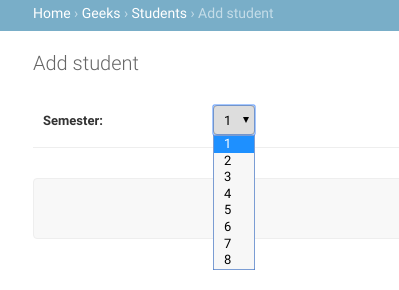# How to use Django Field Choices ?

• Difficulty Level : Expert
• Last Updated : 03 Nov, 2019

Django Field Choices. According to documentation Field Choices are a sequence consisting itself of iterables of exactly two items (e.g. [(A, B), (A, B) …]) to use as choices for some field. For example, consider a field semester which can have options as { 1, 2, 3, 4, 5, 6 } only. Choices limits the input from the user to the particular values specified in `models.py`. If choices are given, they’re enforced by model validation and the default form widget will be a select box with these choices instead of the standard text field. Choices can be any sequence object – not necessarily a list or tuple.

The first element in each tuple is the actual value to be set on the model, and the second element is the human-readable name.
For example,

```SEMESTER_CHOICES = (
("1", "1"),
("2", "2"),
("3", "3"),
("4", "4"),
("5", "5"),
("6", "6"),
("7", "7"),
("8", "8"),
)
```

Let us create a choices field with above semester in our django project named geeksforgeeks.

 `from` `django.db ``import` `models`` ` `# specifying choices`` ` `SEMESTER_CHOICES ``=` `(``    ``(``"1"``, ``"1"``),``    ``(``"2"``, ``"2"``),``    ``(``"3"``, ``"3"``),``    ``(``"4"``, ``"4"``),``    ``(``"5"``, ``"5"``),``    ``(``"6"``, ``"6"``),``    ``(``"7"``, ``"7"``),``    ``(``"8"``, ``"8"``),``)`` ` `# declaring a Student Model`` ` `class` `Student(models.Model):``      ``semester ``=` `models.CharField(``        ``max_length ``=` `20``,``        ``choices ``=` `SEMESTER_CHOICES,``        ``default ``=` `'1'``        ``)`

Let us check in admin panel how semester is created.One can also collect your available choices into named groups that can be used for organizational purposes:

```MEDIA_CHOICES = [
('Audio', (
('vinyl', 'Vinyl'),
('cd', 'CD'),
)
),
('Video', (
('vhs', 'VHS Tape'),
('dvd', 'DVD'),
)
),
('unknown', 'Unknown'),
]
```

The first element in each tuple is the name to apply to the group. The second element is an iterable of 2-tuples, with each 2-tuple containing a value and a human-readable name for an option. Grouped options may be combined with ungrouped options within a single list (such as the unknown option in this example).
For each model field that has choices set, Django will add a method to retrieve the human-readable name for the field’s current value. See `get_FOO_display()` in the database API documentation.

My Personal Notes arrow_drop_up